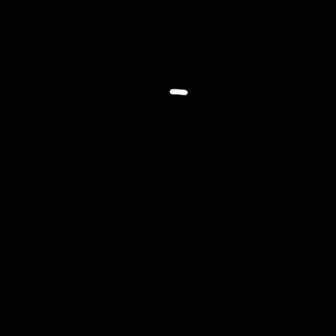# Processing笔记03—基本图形绘制#### 1. 坐标### 2. 基本图形

• 直线：line(x1, y1, x2, y2)
• 三角形：triangle(x1, y1, x2, y2, x3, y3)
• 四边形：quad(x1, y1, x2, y2, x3, y3, x4, y4)
• 矩形：rect(x, y, width, height)
• 椭圆：ellipse(x, y, width, height)
• 扇形：arc(x, y, width, height, start, stop)
``````line(10, 10, 100, 20);
triangle(160, 40, 180, 10, 200, 30);
quad(40, 30, 90, 50, 90, 80, 20, 70);
rect(150, 60, 50, 40);
ellipse(50,120,40,30);
ellipse(130,120,40,40);
arc(40, 150, 50,60,0,3);``````### 3. 绘图顺序### 4.绘图属性

• 描边粗细样式：strokeWeight()，默认参数为1像素
``````size(480, 120);

ellipse(35,60,60,60);
strokeWeight(5);
ellipse(105,60,60,60);
strokeWeight(8);
ellipse(175,60,60,60);
strokeWeight(20);
ellipse(260,60,60,60);``````• 描边端点样式：strokeCap()，参数ROUND(圆形)、SQUARE(方形)、PROJECT(混合)
``````size(480, 120);

strokeWeight(20);
line(30,25,100,95);
strokeCap(SQUARE);
line(130,25,200,95);
strokeCap(PROJECT);
line(230,25,300,95);
strokeCap(ROUND);
line(330,25,400,95);``````• 线段转角样式：strokeJoin()，参数ROUND(圆形)、BEVEL(斜切)、MITER(斜接)
``````size(480, 120);

strokeWeight(10);
rect(20,25,60,60);
strokeJoin(ROUND);
rect(100,25,60,60);
strokeJoin(BEVEL);
rect(180,25,60,60);
strokeJoin(MITER);
rect(260,25,60,60);``````### 5. 颜色

``````size(480, 120);

noStroke(); //隐藏描边
background(0, 25, 50);
fill(255, 0, 0);
ellipse(120, 90, 200, 200);
fill(0, 255, 0);
ellipse(228, -8, 200, 200);
fill(0, 0, 255);
ellipse(256, 126, 200, 200);``````fill()strock()函数中可设置第四个可选参数alpha，用来控制透明度，取值范围0-255。